```
========================================================================
Cybernetics in the 3rd Millennium (C3M) Volume 10 Number 3, Nov. 2012
Alan B. Scrivener — www.well.com/user/abs — mailto:abs@well.com
========================================================================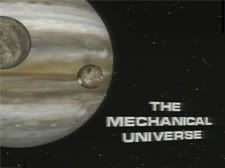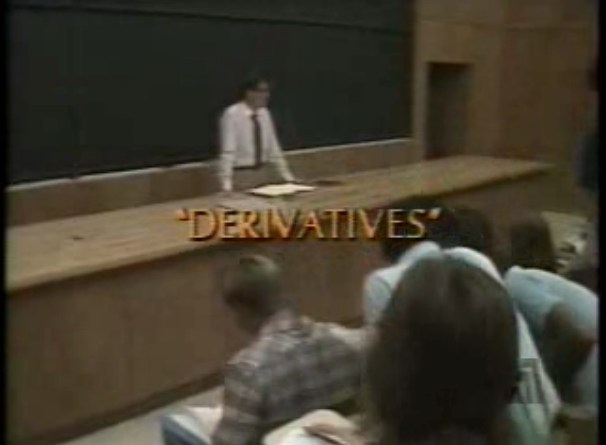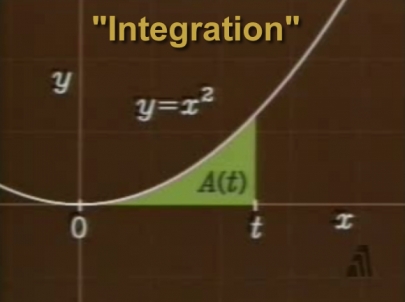screen shots from Caltech's "The Mechanical Universe"
en.wikipedia.org/wiki/The_Mechanical_Universe

In this issue:

Short Subjects
Teaching Calculus to a Sixteen-Year-Old
Note Re: "If It's Just a Virtual Actor, Then Why Am I Feeling Real Emotions?"

Short Subjects: Bateson Documentary in LA;
WELL Update; 'Steps...' On-line; Reading ListBateson Documentary in LA
SHORT NOTICE!  Posted on Nora Bateson's Facebook page yesterday:
From: Earl Crabb <esoft@well.com>
For my friends in the Los Angeles area... Nora Bateson provides a screening
of her film -- An Ecology of Mind -- a film about her father Gregory Bateson
and the relevance of his thought to our day.

Monday, November 5, 2012 at 7:00 p.m.
Art Center College of Design
Hillside Campus - Los Angeles Times Media Center
1700 Lida Street, Pasadena, CA 91103

After the film screening, she will be there for a discussion and Q&A session.
More like Q&Q.. I am alergic to answers.

WELL Update
I got this email recently:
From: Earl Crabb <esoft@well.com>
Subject: The WELL now owned by The Well Group, Inc.
Date: September 20, 2012 4:58:02 PM PDT
To: Alan Scrivener <abs@well.com>

Dear abs,

We did it!  We now own The WELL -- lock, stock and domain name.

The joint press release with Salon is posted in the news conference. In
addition, there is a topic, also in the news conference, for questions and
answers. For you, things should operate as they always have, but if you
discover any issues, please send email to helpdesk@well.com, just as always.

How did this happen? It was the unbelievable show of member support, plus the
member pledges, that empowered the investor group of WELLperns to make the
offer accepted by Salon Media Group. It was Salon that sold us to us, because
keeping this community together was a priority to them. Now, every single
member of the WELL can take a bow, pop the champaign, and celebrate.

You will not see any changes to the service that you currently receive (a
"seamless transition" as the suits would say).

But first let's take a moment and please accept my gratitude. We couldn't have
done it without all of us. Thank you!!  And Whoo-hoo!

Earl Crabb
CEO, The Well Group, Inc.

So it looks like I can keep my email address and web site until the next disruption.
Thanks for the support.
'Steps...' On-line
Not sure how or why, or the legality, but a full PDF copy of Gregory Bateson's
"Steps To An Ecology of Mind" has appeared on-line at Scribd.

( www.scribd.com/doc/58544877/Gregory-Bateson-Steps-to-an-Ecology-of-the-Mind )burning book cake from
butterybooks.com
"You don't have to burn books
to destroy a culture. Just get

(in honor of his passing this year, the new HTTP error code 451 was
introduced, for items blocked by government censorship)

Since our last issue, I found another four books with bookmarks in them:

Rafell, Burton [trans.] Sir Gawain and the Green Knight (c. 1375-1400)

( www.amazon.com/exec/obidos/ASIN/0451626249/hip-20 )

Huxley, Aldous Crome Yellow (1921)

( www.amazon.com/exec/obidos/ASIN/1466285397/hip-20 )

Von Foerster, Heinz [ed.] Cybernetics of Cybernetics (1974)

( www.amazon.com/exec/obidos/ASIN/0964704412/hip-20 )

Hannum, Robert C. and Cabot, Anthony N. Practical Casino Math — Second Edition (2005)

( www.amazon.com/exec/obidos/ASIN/0942828534/hip-20 )

I started and finished two books (including an audio book):

Lewis, Michael Next: The Future Just Happened (2001)

( www.amazon.com/exec/obidos/ASIN/0393323528/hip-20 )

Girard, Joe Joe Girard Complete Audio Box Set CD (2006)

( www.amazon.com/exec/obidos/ASIN/006087709X/hip-20 )

and I launched into three more books:

Collins, Jim Good to Great: Why Some Companies Make the Leap... and Others Don't (2001)

( www.amazon.com/exec/obidos/ASIN/0066620996/hip-20 )

De Kehoe, Joe The Silence and the Sun: An Historical Account of the People, Places
and Events on Old Route 66 and Railroad Communities in the East Mojave Desert, California (2007)

( www.amazon.com/exec/obidos/ASIN/097938270X/hip-20 )

Borneman, Walter R. Rival Rails: The Race to Build America's Greatest Transcontinental Railroad (2010)

( www.amazon.com/exec/obidos/ASIN/140016768X/hip-20 )

Teaching Calculus to a Sixteen-Year-Old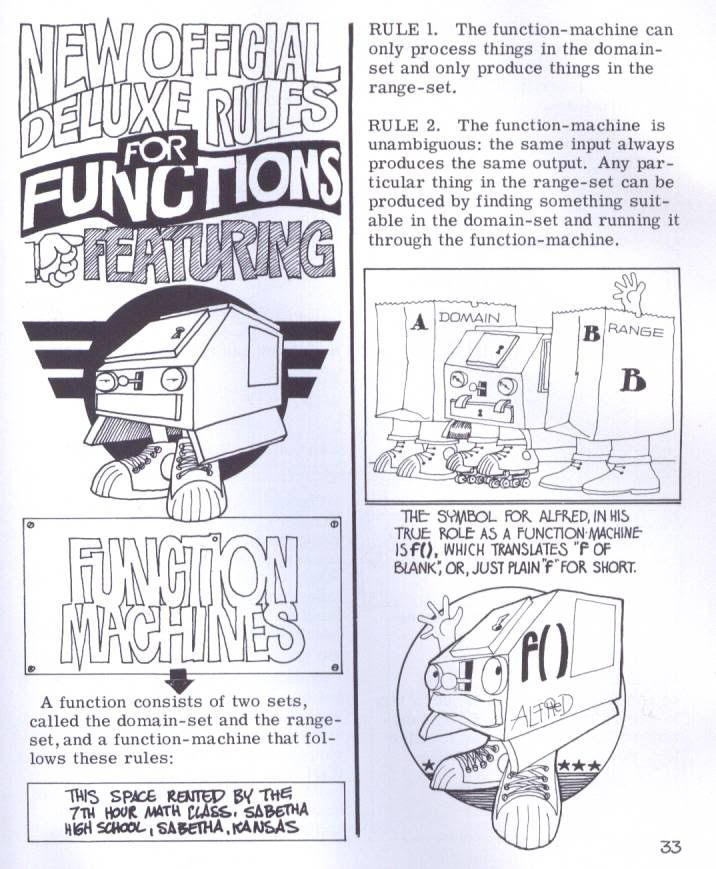a page from "Prof. E McSquared's Calculus Primer"

( www.amazon.com/exec/obidos/ASIN/0971462402/hip-20 )

"And what is the use of a book," thought Alice,
"without pictures or conversation?"

— Lewis Carroll, Alice in Wonderland

On several occasions I've bragged that I could teach calculus to middle school
students, as long as I could skip limits and avoid proofs, and could use computers.

(I especially wanted to avoid limits because I think the fundamental math behind them
is vaguely fraudulent, not unlike the Induction Theorem, and I've found these kind
of shenanigans always cause some students to drop out of math, though they usually
can't articulate why.)

Two summers ago my daughter, then 16, called my bluff.  She wasn't taking summer
school for the first time in a while, and asked me to teach her calculus, so I put
together 11 lessons over about 7 weeks.  I'd say it was a success, and I've since
been asked to reveal my curriculum, so here goes.

First I should say a few words here about how I learned math, and how I taught our daughter.
How I Learned MathI was always bored in school, so I would often sit and read.  The school library
set up a little annex at the back of the classroom, so I had some pretty okay
books.  I loved books on magic, puzzles and tricks, and math puzzles and recreations.
I managed to stay ahead of the math curriculum by reading.  As a result, nothing
in math class was ever a complete surprise to me.  It all made more sense if I'd
encountered it before.  I'm certain this was instrumental in my high grades and
test scores in math.

This momentum did not break down until I was a freshman in college, and exposed
to the temptations of hitch-hiking to Oregon to hang out with some way cool friends
who ate great organic food and lived in this big house that had a belfry, plus an
old couch and a bunch of old National Lampoons on the front porch.  When they
found out I had never been drunk they broke out the frozen daiquiri kit and some
very interesting things happened.  But I digress.  When I returned from this jaunt I took
a Caluclus 3 final and encountered the Del operator on the test, which I had
never laid eyes on before.

( en.wikipedia.org/wiki/Del_operator )

It looked like an upside-down triangle.  I was staring at stuff like this in panic: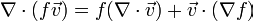For the first time in my life, I began to appreciate what the kids with math anxiety
had been experiencing all the way through school, staring at a test with no clue how
to proceed.

I also saw how the whole math experience could get into a tailspin, with anxiety
causing poor performance causing anxiety in a destructive positive feedback loop.
How I Taught Our DaughterWhen I was in high school I started a binge of reading books on advances in
education, and I soon came to the delightful books by James Herndon.  His
How to Survive in Your Native Land (1971)

( www.amazon.com/exec/obidos/ASIN/0867094087/hip-20 )

is an affable manifesto on what it looks like is wrong with education from a
teacher's point of view.  At one point he described a survey article he read by
some researchers who looked at all the ways scientists had tried to increase the
intelligence of rats, and which ones had worked.  Their conclusion was that the
only reliable way to increase the intelligence of rats was to let them roam freely
in a complex and varied environment.

This became my main educational strategy.  Our house was always filled with the
best educational stuff we could find, and we never made her play with any of it.
It was just there, and sometimes we'd make helpful suggestions when she was bored.

We made sure the house had several computers, PCs and MAcs, with games she would
enjoy, and over time she became expert at troubleshooting common comuputer
problems.  I also made it a point to track educational games and pasttimes,
especially when recommended by Martin Gardner

( en.wikipedia.org/wiki/Martin_gardner )

or Raymond Smullyan.

( en.wikipedia.org/wiki/Smullyan )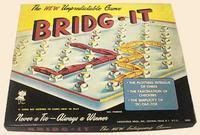Bridg-It game shown at Boardgame Geek
boardgamegeek.com/boardgame/11052/bridg-it

For example, Gardner recommended an old board game from the 1960s called
"Bridg-It," originally invented by communications theory pioneer Claude Shannon.
I found one on eBay.  It was fun at parties.

( en.wikipedia.org/wiki/Shannon_switching_game )

To help our daughter avoid math anxiety, I made up a series of math games for
her to play starting about age 3.  I don't have room to detail them here,
but one day I hope to write a book about this called "How To Raise a Genius
in Ten Easy years."

This approach worked very well; she has always done well in math.  (She did tell
me as a pre-teen that there were actually 2 kinds of math, the fun daddy math
and the boring school math.)

Another lesson I learned from Herndon was "make them take it away from you."
In one of his books he describes how he got his middle school students to make
a film.  He showed up at school with a camera and started shooting.  He wouldn't
explain and he wouldn't share.  They finally dragged it out of him that he'd been
loaned the camera and given the film stock so he could use them in his teaching.
They figured that meant he shoud let the kids participate, and pretty soon they
had a plan "for the movie" and eventually they kicked him off the project.

This worked smashingly for us.  For example, my daughter begged me to let her play
with iMovie on my old clamshell Mac, and when I finally gave in she became an
accomplished editor in no time.

So, naturally, I was ecstatic that she wanted calculus lessons.  It may have been
a high point of my life.  But I played it cool.  I prepared about an hour of
material for each lesson, and told her when I had it ready, and then I waited for her
to tell me when she wanted the session.  There was no pressure, and everything was
voluntary and on-demand.

By way of background, I should mention that she had completed Algebra I and II
in the California high school curriculum.
Lesson 1 — 24 June 2010
At the beginning I told her that I was going to follow the approximate sequence
of material in a previous issue of C3M, volume 6 number 1, "Everything Has To Go
Somewhere ~ OR ~ Eigenvectors and You" from January 2007, in case she wanted
to refer to it during the class or later on.

( www.well.com/user/abs/Cyb/archive/c3m_0601.html )

Next I drew a little diagram to show how math has been traditionally done
through ages, contrasted with how it needs to be now that we have cheap computers.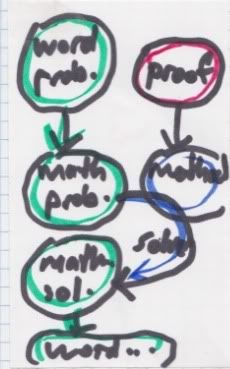In the old days things worked like this: Mathemeticians would create one or more
proofs, which enable methods for solving problems, as shown by the red and
blue circles on the right side.  Later, someone cames along with a problem, initially a
word problem, and they proceeded to convert it to a math problem, then use the
method to solve the problem, then convert the math solution into a word solution.
In educating students, we taught them the proofs so they'd be well-rounded, or
something, as well as the methods so they could solve problems.

Well now you don't need the proofs or the methods, I explained.  Symbolic solvers
like Mathematica from Wolfram Research

( www.wolfram.com/mathematica )

can solve most the equations anyone will ever encounter, so we can concentrate on
translating between words and math and back.  But we still need to understand
the concepts!

Here is the roadmap I laid out.  I planned to cover: differential calculus
integral calculus
mechanics
Ordinary Differential Equations (ODEs)
Finite State Machines (FSMs)
systems theory

Here are the resources I planned to use (* = didn't use after all):

Mechanical Universe

textbook, 1986, by Steven C. Frautschi, Richard P. Olenick, Tom M. Apostol, David L. Goodstein *

( www.amazon.com/exec/obidos/ASIN/0521304326/hip-20 )

videos, 1985, by Dr. David Goodstein, Jim Blinn, Peter F. Buffa, Mark Rothschild

( www.amazon.com/exec/obidos/ASIN/B0027K9UES/hip-20 )

( www.learner.org/catalog/series42.html )

( www.phys.ufl.edu/demo/demobook/MechUniv/MechU.html )

Prof. E McSquared's Calculus Primer (comic book, 1974) by Swann Howard

( www.amazon.com/exec/obidos/ASIN/0971462402/hip-20 )

An Introduction to Cybernetics (book, 1966) by Ross Ashby

( www.amazon.com/exec/obidos/ASIN/B001TC7UVA/hip-20 )

Excel spreadsheet software from Microsoft Corp. *

( www.amazon.com/exec/obidos/ASIN/B0039L2XG6/hip-20 )

GNU Octave free software (Matlab work-alike) from the Gnu Foundation *

( www.gnu.org/software/octave )

the Application Visualization System version 5 (AVS5) software from Advanced Visual Systems Inc. (AVS Inc.)

( www.avs.com/products/avs5/index.html )

the C programming language

( en.wikipedia.org/wiki/C_programming_language )

the Collosal Cave text-based Adventure game

the Wikipedia collaborative encyclopedia

( en.wikipedia.org )

the Wolfram Alpha web site, which provides an augmented front-end to Mathematica

( www.wolframalpha.com )

the text-based units program on many UNIX systems

( www.gnu.org/software/units )

the text-based basic calculator (bc) on most UNIX systems

( linux.die.net/man/1/bc )

Isaac Asimov's science and math essays from Fantasy and Science Fiction (S&SF) *

( www.asimovonline.com/oldsite/Essays/f_and_sf_essays.html )

( www.amazon.com/exec/obidos/ASIN/0671821342/hip-20 )

Ralph Abraham and C. D. Shaw's so-called "chaos comix," Dynamics, the Geometry of Behavior (1992)

( www.amazon.com/exec/obidos/ASIN/0201567172/hip-20 )

( www.aerialpress.com/DYN/DYN4Pt1Ch0X.pdf )

To begin we watched Mechanical Universe Epsiode 1: "Introduction" (28:52) and discussed it.

For homework I gave her blank 3x5 cards and a calligraphic pen, plus a print-out of the
upper and lower case Greek alphabet, and had her create Greek letter flashcards.  I explained
that in my many years of math tutoring I found legibility to be one of the biggest issues
in succeeding.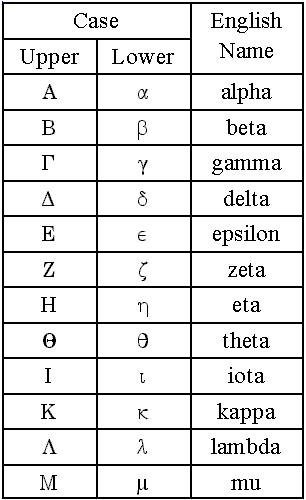Lesson 2 — 25 June 2010
concepts: constant acceleration

( en.wikipedia.org/wiki/Constant_acceleration#Uniform_acceleration )

We watched Mechanical Universe Epsiode 2: "The Law of Falling Bodies" (29:06) and discussed it.

This episode included footage of Apollo 15 astronaut David Scott on the moon, using a
feather and a hammer to prove that Galileo was right about objects of different masses
falling at the same acceleration in a vaccuum.
Lesson 3 — 28 June 2010
concepts: function

( en.wikipedia.org/wiki/Function_%28mathematics%29 )

transform

( en.wikipedia.org/wiki/Transform_%28mathematics%29 )

derivative

( en.wikipedia.org/wiki/Derivative )

When my daughter was maybe eight I used to play a game with her I called "the
function game."  She would give me a number and I'd give her back a number, and
after a few examples she'd try to guess the rule.  Here is a typical exchange
(F=father, D=daughter):
D: 1
F: 2
D: 2
F: 4
D: 3
F: 6
D: It's always twice as much!

D: 1
F: 7
D: 2
F: 7
D: 3
F: 7
D: It's always seven!

D: 1
F: 1
D: 2
F: 4
D: 3
F: 9
D: I don't know.
F: It's the number squared, you know, the number times itself.
D: Right!
And so on.

Also, they pretty thoroughly covered functions in her algebra classes, defining
them in terms of set theory, Domain and Range and all that.  So we moved on to the
concept of a "transform."

"Prof. E. McSquared's Calculus Primer" was helpful in this regard.  It showed a
function as a robot that took in one number and put out another.  By analogy
a transform was a different kind of robot that took in one function robot
and put out another.  It had a rule for transforming one function into another.

I then went to explain that a "derivative" was a special transform that always
gave the slope of a function at each point — another concept she was
familiar with.  I followed with examples:

As we saw, Galileo observed that an object near the surface of the Earth, without too
much air resistance, will fall a distance proportional to time squared.  Using feet
and seconds, we have position p given by:

p(t) = 16*t^2 ft

The derivative or rate of change of p(t) is velocity, a linear function of time t:

v(t) = p'(t) = 32*t ft/sec

(In reading this notation aloud we say "p prime.")

The second derivative of p(t), also the derivative of v(t), is the acceleration
of the object at time t, which is a constant.

a(t) = v'(t) = p''(t) = 32 ft/sec^2

The I drew a graph much like this one to show all three, and how each derivative was
the slope of the function it was applied to.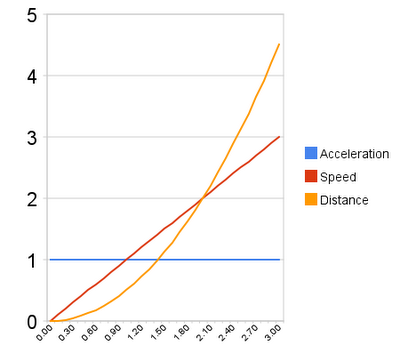illustration for Betsy's Devine Algebraic Limerick,
from pergelator.blogspot.com

I told her there are Differentiation Rules for doing that transform we call differentiation,
and showed her the polynomial or elementary power rule as an example.

( en.wikipedia.org/wiki/Differentiation_rules )

This was how, for example, we took the derivative of 16*t^2 and got 32*t.

Next I showed how we could use the UNIX programs basic caluclator (bc) and units
to solve some simple problems for us.  Invoking bc with its math library (what I typed is
in green):
% bc -l
bc 1.06
Copyright 1991-1994, 1997, 1998, 2000 Free Software Foundation, Inc.
This is free software with ABSOLUTELY NO WARRANTY.
For details type `warranty'.
scale=4
16*.1*.1
.1600
16*.5*.5
4.0000
16*1.5*1.5
36.0000
^D
%
So what I've done there is compute — to four decimal places — how far an
object falls after 0.1 second, 0.5 seconds, and 1.5 seconds.  This can also be easily
scripted in a shell.

( www.dreamsyssoft.com/unix-shell-scripting/intro-tutorial.php )

I also showed her the "units" program for converting physical units.  For example:

% units
586 units, 56 prefixes
You have: 14000 feet/second
You want: miles/hour
* 9545.4545
/ 0.0001047619
You have: ^D
%
Here I've let the computer figure out that 14,000 feet/second is approximately 9,545.4545
miles/hour.  (It also gives the inverse in case you need it.)

Next we watched Mechanical Universe Epsiode 3: "Derivatives" (28:53) and discussed it.

She wanted some homework, so I assigned some problems on computing derivatives by letting
the value of "h" vanish in f(a+h)-f(a)/h: Prof. E McSquared's Calculus Primer, Chap III, 4 probs.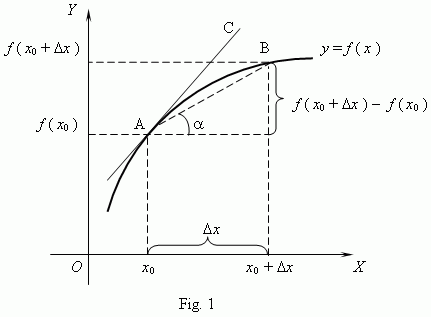definition of differentiation illustrated at
www.splung.com/content/sid/1/page/differentiation
Lesson 4 — 29 June 2010
concept: computer algebra system (symbolic solver)

( en.wikipedia.org/wiki/Symbolic_solver )

When daughter asked for the next lesson she revealed that she'd had less enthusiasm
than she expected for the homework.  She's only done one problem, and didn't want
to do any more.  Fair enough; I worked around this.

I read to her from C3M, volume 6 number 1, "Everything Has To Go Somewhere ~ OR ~
Eigenvectors and You" from January 2007, the sectioned titled "CALCULUS WITHOUT
PROOFS IN THE DIGITAL AGE"

( www.well.com/user/abs/Cyb/archive/c3m_0601.html )

Then I showed her how she didn't need to know the rules for differentiation in
the 21st Century.  Wolfram Alpha will do all that now.

( www.wolframalpha.com )

I brought up the web site and typed:
y(x) = x^3 - cos(x)
into Wolfram Alpha and it told me the derivative is
3 x^2 + sin(x)
as well as some other fascinating stuff.

( www.wolframalpha.com/input/?i=y%28x%29+%3D+x^3+-+cos%28x%29 )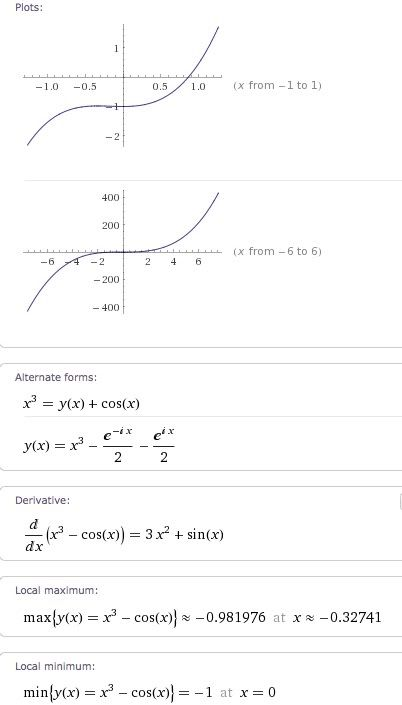I think we watched Mechanical Universe Epsiode 4: "Inertia" (28:36) and discussed it.

Lesson 5 — 2 July 2010
concepts: utility function

( en.wikipedia.org/wiki/Utility_function )

minimum and maximum

( en.wikipedia.org/wiki/Minimum )

vectors

( en.wikipedia.org/wiki/Vector_%28mathematics_and_physics%29 )

At this point I wanted to show my daughter how this stuff could actually be used to solve
real problems, for example in business.  In what they call management science, and
used to call operations research, there are a whole family of problems that involve
defining a utility function and then minimizing or maximizing it.

I drew a crude diagram to illustrate a problem involving locating a factory along a ten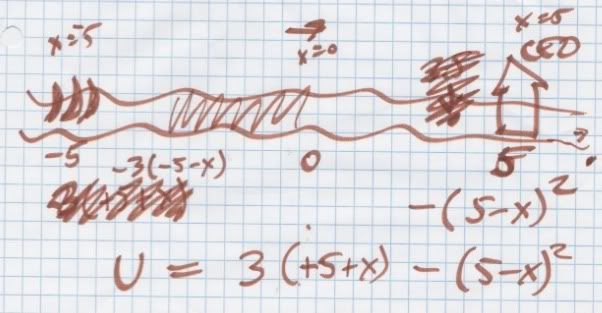Variable x the thing we have control over, in this case the position of the factory
along the road from -5 to 5, and the utility function u(x) represents dollars in revenue
we would like to maximize, or dollars in cost that we would like to minimize.  In this
example it is a cost.  We start with the information that each mile away from the river
results in three units of cost (such as maintaining a pipeline per mile), while the distance
to the CEO's house in miles, squared, gives units of cost for his travel logistics.

In this coordinate system, the plant distance to river = x + 5, while plant distance to
CEO = -x + 5.  This gives us a utility function of:
u(x) = 3*(5+x) + (5 - x)^2

Using algebra to reduce (or using Wolfram Alpha) we get:
u(x) = x^2 - 7x + 40
Now we can use our newly-learned calculus to find the minimum cost.  First we find
the derivative of u(x):
u'(x) = 2x - 7
Then we set x=0, to find the places where a maximum or minimum occur.  Think about it:
the rate of change is always zero, and the slope is always zero, at the top of a hill
or the bottom of a valley.

The only trick is making sure you really have a minimum, not a maximum.
In the old days we would draw the graph, or try a few nearby values of u(x),
but now Wolfram Alpha will do the whole thing for us.

( www.wolframalpha.com/input/?i=u%28x%29+%3D+3*%285%2Bx%29+%2B+%285+-+x%29^2 )

Conclusion: at x = 7/2 (3.5), the minimum cost is 111/4 (27.75).  Build the factory at
x = 3.5 miles, a mile and a half from the CEO's house.

Then watched Mechanical Universe Epsiode 5: "Vectors" (28:51) and discussed it.

I told her that the mathematics of vectors was around a lot longer than modern vector
notation.  I showed he a picture of Oliver Heaviside, who gave us the letter with the
arrow over it, instead of strings of near-identical equations.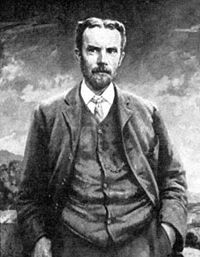Oliver Heaviside picture
from Wikipedia commons

( en.wikipedia.org/wiki/Heaviside )

I explained that Maxwell's famous equations of electrodynamics wouldn't fit on a tee shirt
if it had't been for Oliver; we'd need a bed sheet.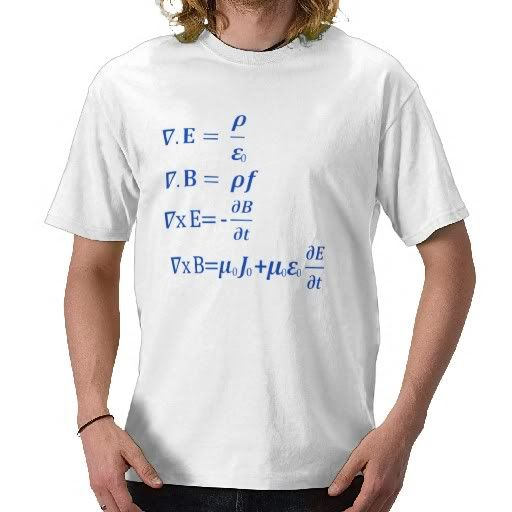Maxwell's Equations shirt from
www.zazzle.com

(Notice that insidious del operator.)
Lesson 6 — 29 June 2010
concepts: series of differences between squares

( betterexplained.com/articles/surprising-patterns-in-the-square-numbers-1-4-9-16 )

Babbage's difference engine

( en.wikipedia.org/wiki/Difference_engine )

Taylor series

( en.wikipedia.org/wiki/Taylor_series )

ordinary differential equations (ODEs)

( en.wikipedia.org/wiki/Ordinary_differential_equations )

I shared with daughter how I'd discovered a pattern in the square numbers as a kid,
not knowing it was already well understood by mathematicians: the difference between
each square number (i.e., a whole number squared) and the next gives the series which
we know as the odd numbers.  Below I have written a series of numbers squared, listed
vertically, starting with 0^2 = 0, then 1^2 = 1, 2^2 = 4, 3^2 = 9, and so on.  In the
next column I have written the difference between each square and the next: 1, 3, 5,
and so on.  It the next column are differences between those numbers, which are
all two: each odd number differs from the next by 2.  And in the last column are the
differences between each 2 and the next, obviously a series of 0s.
0
1
1     2
3     0
4     2
5     0
9     2
7     0
16     2
9     0
25     2
11    0
36     2
13
49

Of course, if you continued the columns to the right they'd be all zeros, since 0 - 0 = 0.
What I didn't realize as an experimenting kid is that this pattern obtains for all polynomial
expressions with a single integer variable.  How many columns you can subtract before
you hit the zeros is a function of the degree of the polynomial.

This is the principle behind the Difference Engine designed by Charles Babbage in
the 1820s.  (More on this later.)

I showed daughter the cover of the steampunk novel "The Difference Engine" for
a visual reference.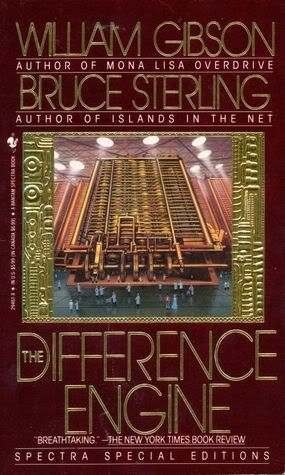cover illustration from "The Difference Engine"
(novel, 1991) by Gibson & Sterling

( www.amazon.com/exec/obidos/ASIN/0440423627/hip-20 )

I told her how I had a delightful physics professor at UCSC named Dorfman,
who was South African and had a clipped British-esque accent.  One day he asked
us if we kenw what a Taylor Series was.  We'd all studied the proof of the
Taylor Series in the prerequisite calculus classes, but nobody understood it.
He was aghast.  "What are they teaching you?" he muttered.  He took a 20-minute
detour and gave us a proof-less explanation of what it meant and what it
was for.  And it was this intuition that I wanted to pass on to me daughter.

Consider a problem that Norbert Weiner studied a lot, when he was inventing
cybernetics, function prediction.  Let's say you've got a series of
numbers coming down a pipe at regular intervals, like readings from a temperature
sensor, or stock purchse prices from the NASDDAQ.  Given a series of numbers,
how do you predict the next one?

ZERO ORDER

One simple solution is to always guess zero.  It's probably going to be wrong
most of the time, but it will also probably have an error that stays within
a stable range.  It's also very inexpensive to compute.

FIRST ORDER

A better approximation is to guess a contstant, C, picked by some reasonable
process like the last value, or an average of previous values.  It will also
probably be wrong, but have a stable error range.

SECOND ORDER

If you look at the last two values and subrtact them, and get a difference
(often called delta), and then add the delta to the last value to
apply the change again, this gives an even better guess.

HIGHER ORDERS

By taking differences of differnces and finding rates of rates of change,
etc., the order of approximation can be raised and often improved.  (Of course,
for every function prediction scheme there are toxic functions that mess it up.)

If you take this descrete example and generalize it for a coninuum, you get
approximations of the form:

0: y = 0
1: y = C
2: y = Ax + C
3: y = Ax^2 Bx + C
etc.

The Taylor series expoits this almost magical ability of polynomials to
approximate continuous functions.  The actual formula given by Taylor is:general formula for Taylor series

Note that what looks like f raised to the (n) is meant to express the nth derivative
of f(x), i.e., f super (0) is just f, f super (1) is f', f super (2) is f'', etc.
So if you can find all the derivatives up to infinity at any point x0, you can
approximate a function.  (When x0 is taken to be 0, you get the simpler, older
dicovery, the Maclaurin Series.)

Let's see it in use to approximate a sine wave, which is function with a well-known
value at 0: sin(0) = 0.  It also has well-known derivatives, being its own 4th
derivative, so they can be easily computed to arbitrary sized derivatives.

From calculus of trig functions we get:

derivative of sin(x) is cos(x)
derivative of cos(x) is -sin(x)
derivative of -sin(x) is -cos(x)
derivative of -cos(x) is sin(x)

also: sin(0) = 0 and cos(0) = 1.

Plugging these into the Taylor Series we get:

IN OUT
f(0)    =  0 f(x) = 0
f'(0)   =  1 f(x) = x
f''(0)  =  0 f(x) = x
f'''(0) = -1 f(x) = x - x^3/3

Here is an illustration of the sucesive approximations of a sine wave this way
(black = actual sin function; colors red through violet represent increaing order):succesive approximations of sin(x) with Taylor series, from Wikipedia

Each higher-order term adds more "curviness," as needed.

The technique of approximation with polynomials using Taylor series is fundamental
to doing real calculations, such as generating trig tables.  In the age of cannon
warfare, armies used to draft mathematicans to compute ballistics tables this way.

I also gave her a first look at ordinary differential equations (ODEs).
I explained that it could be viewed as a guessing game.  What function has a
derivative of 0?  And so on.  Here are a list of single equation, single variable
ODEs and their solutions.  (Note that, just to mess you up, these are often written
as x being a function of t — x(t) — instead of the more familiar y(x) or
f(x) forms).

problem solution
x'(t)    = 0 x(t) = C
x'(t)    = 1 x(t) = t + C
x'(t)    = x x(t) = e^t + C
x''''(t) = x x(t) = sin(t + B) + C

Note that the third row provides a concise alternative definition of e and the
fourth row provides an alternative definition of a sinusoidal function.

I explained it was also possible for pairs of equations to have pairs of variables
just like in algebra.  This field of math is sometimes called linear algebra,
when the equations are linear, and solvable with classical analytics.

For example:

x' = x + y
y' = x - y

would be a pair of ODEs with two variables.  A closed form solution
would then give x and y in terms of t.

Wolfram Alpha gives the solution, which are two extremely gnarly expressions
for x(t) and y(t).

( http://www.wolframalpha.com/input/?i=++++x%27+%3D+x+%2B+y+%3B++++y%27+%3D+x+-+y )

Most of what we call "hard science" involves writing rules as ODEs and then solving
them somehow.

Lastly we watched Mechanical Universe Epsiode 6: "Newton's Laws" (29:08)
and discussed it.

Lesson 7 — 31 July 2010
concepts: integral

( en.wikipedia.org/wiki/Integral )

fundamental theorem of calculus

( en.wikipedia.org/wiki/Fundamental_theorem_of_calculus )

Newton's and Leibniz's notations

( en.wikipedia.org/wiki/Newton%27s_notation )

( en.wikipedia.org/wiki/Leibniz%27s_notation )

I began this lesson by talking in a little more detail about how the difference
engine works.  Previously I explained how the diference of squares were odd numbers,
the difference of odd numbers was always 2, and the difference of any pair of 2s is 0.
(And of course 0 - 0 = 0, so it's "zeros all the way down" after that.)  But, who
needs a register full of 0s?  The difference engine actually runs in reverse, and
so it doesn't find differences; it integrates as we say now.  Starting with a
column of 2s on the left, placing a 1 atop the middle column and adding
it to the 2 on its lower left, gives us 3, which we write below.  Then 2 + 3 = 5,
2 + 5 = 7, etc., generating the second column.  The third column is made by starting
with a 1 at the top, adding the 3 on its lower left to get 4, then adding 5 to that
4 to get 9, and so on, generating a column of the squares, like so:

1
2     1
3
2     4
5
2     9
7
2     16
9
2     25
11
2     36
13
2

Of course, a register filled with sequential square numbers is a lot more useful
than a register full of 0s.  And by varying the process, values for any polynomial
(restriced by the size of the machine) can be generated; and with the Taylor Series,
a polynomial can approximate most functions.  Huzzah!

Next I explained the concept of the area under a curve.  A function y of x can
be considered starting at any vertical line (usually x=0) and sweeping out an area
as x increases.  Traditional calculus provides a series of arcane and not always
effective techniques for finding these integral functions in closed form.
Engineers and other ptagmatists will plot the function on graph paper and count
the squares, or even cut out the curve and weight the paper.  Nowadays, of course,
there are computer approximation techniques as well.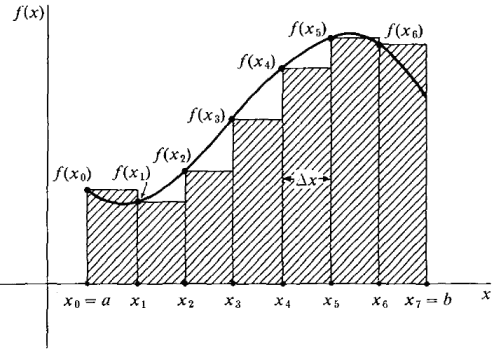illustration of area under a curve from
Virtual Institute of Applied Science (VIAS)
www.vias.org/calculus/04_integration_01_02.html

This area curve, a transform of the original function y(x), is called the indefinite
integral of the function.  The big "aha" in calculus, and one reason we stand in such
awe of Newton, is that he showed that the derivative transform and the (indefinite)
integral transform are the inverses of each other.  A derivative of an integral, or an
integral of a derivative, is the original function (possibly with a constant added).
This is called the fundamental theorem of calculus.

There is also the definite integral, which is just an indefinite integral with the
additon of starting and ending values for x.  It evaluates to a number, the area
under the interval, and not a function.

This tool is massivley useful for finding areas, averages, even volumes (using

One particularly startling result is that the are under the multiplicative inverse
function, y(x) = 1/x, is the log to the base e of x.

integral 1/x dx = ln(x) + c

Wolfram Alpha will verify this.

( www.wolframalpha.com/input/?i=integral+1%2Fx+dx )

This provides another concise definition of e, which I find to be quite a
mind-blower.

At this point I stopped to review the alternate notations provided by Newton
and Leibnitz.  I'd been using the "x prime" style of derivative: x' or x'(t)
all along.  Newton actually started with fluxions, little bits
of flux (the stuff of flux capacitors, perhaps?) that he would write as an x
with a dot above it.  A second dreivative would have two dots, and so on.  It
quickly became a visual mess, though engineers and others still sometimes use
the notation for simple problems.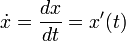Newton's and Leibnitz's notations from Wikipedia

Leibnitz independently invented calculus and started a battle for credit with
Newton that ended up influencing European politics.  The British, clinging
to Newtons fluxions, did a poorer job of aiming their cannons, especially
when up against the French, and finally adopted Leibnitz's notation.  German
philosopher Leibnitz gave us the differentials, little derivative fragments
that seemed nonsesnical when taken alone, but which could be combined according
to rules to solve problems.  The derivative of x(t) is represented:

dx/dt

which is the quotient of two differentials.  We saw above how an integral uses
a differential at the end to indicate the integrating variable.

The second derivative of x(t) is:

d^2x/dt^2

The integral is possibly the most massivley useful tool in math.  It totally
oversolves the problem of finding areas.  Here is a simple example, which the
ancients were rather obsessed with: finding the area of a circle.

We all know a circle is a set of points in the plane equidistant from a center point.
Placing the center at the origin of an XY plane, and letting r be the radius, we can
use the Pythagorean Theorem (which even the Scarecrow knew in the Wizard of Oz)
to write:

x^2 + y^2 = r^2

Solving for y we get:

y = sqrt(r^2 - x^2)

We need to plug in an actual value for r to avoid confusing Wolfram Alpha,
so let's say r = 1.  Now:

y = sqrt(1 - x^2)

( www.wolframalpha.com/input/?i=y+%3D+sqrt%281+-+x^2%29 )

We can see Wolfram Alpha plotting this as a semi-circle.  (The scale is a little
hinky.)  We'll need to double the answer to get the whole circle.  Finding the
integral with respect to x:

integral sqrt(1 - x^2) dx

( www.wolframalpha.com/input/?i=integral+sqrt%281^2+-+x^2%29+dx+ )

The answer is given as approximately 1.5708, or Pi/2, which we then must multiply
by 2.  This gives us...  why is always the same hands?  Pi.

Moving right along, my friend John Z. used to say that the purpose of integral
calculus was to teach undergraduates how to find the rate at which the water level
is rising in an inverted cone water tank being filled at a constant rate.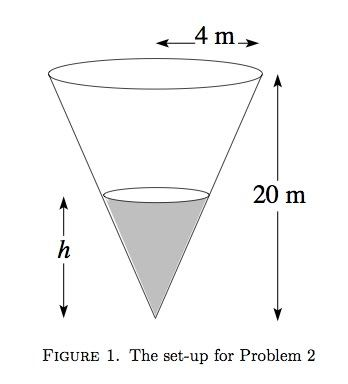The University of Arizon math department web site has a typical version of this
classic problem, number 2A.

Q: A water tank is in the shape of an inverted cone with height 20 m and top
radius 4 m. Water is flowing into the tank at a constant rate of 0.1 m3/min.
How fast is the water level in the tank rising at the moment that the depth
of the water is 5 m?

I pointed out that in the on-line document, the first 3 steps are the "word problem"
and setting up the equation.  You can then let Wolfram Alpha do steps 4 and 5.

After that (or maybe before) I think we watched Mechanical Universe
Epsiode 7: "Integration" (29:08) and discussed it.

Lesson 8 — 2 August 2010
concepts: deterministic finite automaton (finite state machine without inputs)

( en.wikipedia.org/wiki/Deterministic_finite_automaton )

basin of attraction

( en.wikipedia.org/wiki/Basin_of_attraction )

finite state machine with inputs

( en.wikipedia.org/wiki/Finite-state_machine )

This lesson took much of its material from Ashby  (op, cit.) — the
delightful Introduction to Cybernetics.  I began with the concept of a
finite state machine, which can be illustrated by the old prank, a
piece of paper on which is written: "How to keep a fool busy for hours
(see other side)."  On both sides.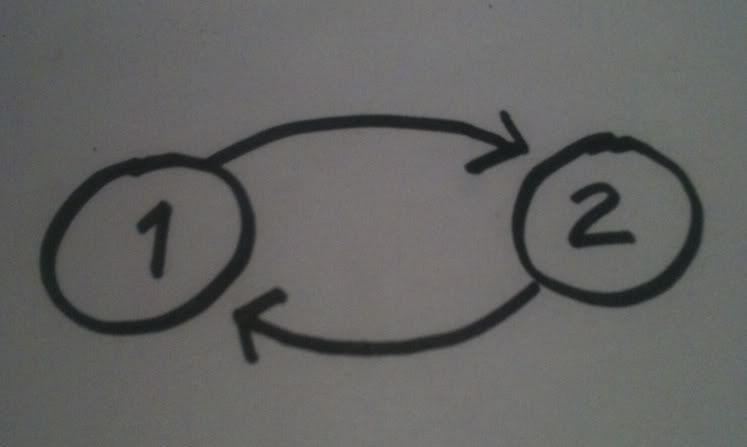2 state FSM drawn by ABS

If we number the states 1 & 2, the output of this machine is:

1 2 1 2 1 2 1 2...

Everybody following along?  Good.  Things get more compicated as you add
states.  Ashby defined the variety of a FSM as a measure of
the number of states it can possibly end up in, and pointed out that over
time the variety always stays the same or goes down.  (Kind of like the
way entropy always stays the same or goes up.)  If an arrow leads out of
state 1 to state 2, but there is no path back through the machine from
2 to 1 (i.e., the transisiton from 1 to 2 is a "trap door"), then once
the transition is made to state 2 the  variety has gone down permanently.

I explained how you can sort all the states in a machine into basins of
attraction, kind of like watersheds.  If you spit in San Deigo County
chances are the water will end up in one of 10 rivers or creeks (San Juan,
Santa Margarita, San Luis Rey, Carlsbad, San Dieguito, Penasquitos, San Diego,
Pueblo, Sweetwater, Otay, Tijuana), fed by the ten basins or watersheds.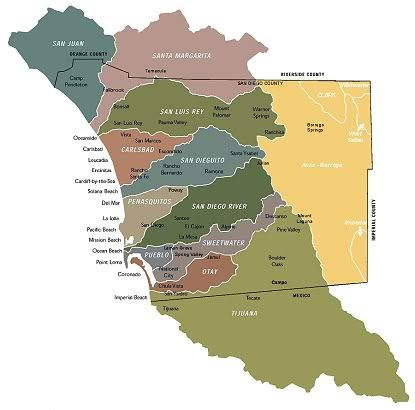San Diego County watersheds from county web site
www.sdcounty.ca.gov/pds/watershed/watersheds.html

Next I explained a finite state machine with inputs, in which each
transition is controlled by an input message, and the machine can be expressed
by a table listing each possible input vs. each state, giving the new state.
For example, consider this simplistic text-based Adventure Game:

input ->initialstate|V OUT INUPDOWN NORTH SOUTH
well house outsidewell house well house * well house * secretbasement well house * outsidewell house
secretbasement secretbasement * well house secretbasement * secretbasement * secretbasement * secretbasement *
outsidewell house outsidewell house * well house outsidewell house * down hill well house down hill
down hill down hill * down hill * outsidewell house lost inwoods outsidewell house lost inwoods
lost inwoods lost inwoods * lost inwoods * lost inwoods * lost inwoods * lost inwoods * lost inwoods *

* = no move

Here is a typical game:

You are in well house.
WEST
You can't go in that direction.
You are in well house.
SOUTH
You are outside well house.
NORTH
You are in well house.
OUT
You are outside well house.
IN
You are in well house.
DOWN
You are in secret basement.
UP
You are in well house.
OUT
You are outside well house.
SOUTH
You are down hill.
SOUTH
You are lost in woods.
NORTH
You can't go in that direction.
You are lost in woods.
UP
You can't go in that direction.
You are lost in woods.
IN
You can't go in that direction.
You are lost in woods.
OUT
You can't go in that direction.
You are lost in woods.
WEST
You can't go in that direction.
You are lost in woods.

a simple C program to let her design simple "mazes" — with objects
but without creatures — simply by editing text files, so she got
the concept immediatley.

In this FSM/maze, the "lost in woods" state is the only attractor, and the
whole machine is its basin.

input ->initialstate|V OUT INUPDOWN NORTH SOUTH
dungeon dungeon * dungeon * dungeon * dungeon * dungeon * dungeon *

Now ther'd be two basins: the dungeon, and everywhere else.  Only if you started
in the dungeon would you end up there.

Lastly we watched Mechanical Universe Epsiode 8: "The Apple and the Moon"
(28:39) and discussed it.  (This was probably the end of our video viewing.)

Lesson 9 — 6 August 2010
concepts: Law of Requisite Variety

( en.wikipedia.org/wiki/Law_of_Requisite_Variety )

attractor

( en.wikipedia.org/wiki/Attractor )

dynamics

( en.wikipedia.org/wiki/Analytical_dynamics )

For this next lesson I drew a diagram of five circles labeled A through F
forming a roughly circular pattern.  I used this as a "game board" to play
a game with daughter that illustrated the concept of requisite variety.

Unfortunately neither she nor I can remember two years later what the game was.
Maybe you can make up your own.

One wsy to think about requisite variety is to imagine that your friend is
a "subsystem" and blurts out a number every ten seconds.  You then may reply
with another number, and the two are added to produce a sum.  You are the
"controller" of the subsystem.  Your goal is to have the sum always equal zero.
Your friend is trying to mess you up.  They say 3, you say -3.  They say -442,
you say 442.  They say 10^38, you say -10^38.  Clearly, for you to keep
succesfully controlling the result, you must be able to name every number your
friend can, but negated.  If they have a greater range or accuracy, they can
toss out a number you can't negate.  This is a form of the Law of Requisite Variety.
The variety of the controller must be at least equal to the variety of the subsystem
being controlled.

Next I taught her a card trick that illustrates the concept of attractors
quite nicely.  It's from the book Penrose Tiles to Trapdoor Ciphers: And the
Return of Dr Matrix (1989) by Martin Gardner.

( www.amazon.com/exec/obidos/ASIN/0883855216/hip-20 )

The supershort version is that you flip through a deck of cards face up one at
a time and have your spectator silently follow these instructions.  Pick any early
card, and use it is a "key card" and count its value (ace = 1, royals = 5) to the
next key card, and repeat until you can't go on.  You write down your predicition,
the spectator names their final card, and 5/6 of the time you are right.  How?  You
simply follow the same instructions you gave the spectator yourself silently.
Chances are you will both end up in the same basin, and therefore at the same
attractor.

Next I read aloud from the first page of the paper A Mathematics for Physiology (1983)
by Dr. Alan Garfinkel, UCLA.

( ajpregu.physiology.org/content/245/4/R455.abstract )

( www.ncbi.nlm.nih.gov/entrez/query.fcgi?cmd=Retrieve&db=PubMed&list_uids=6624944&dopt=Abstract )

It begins:

The revolution that Newton introduced into science had three independent components.
Two belonged to physics: the inverse-square law of gravitation and the idea that
force is proportional to acceleration. The third, and most basic, was mathematical:
the calculus, as the language in which all dynamics is to be formulated.

The essence of the calculus lies in the concept of a differential equation as the
basic form of explanation of physical change. A differential equation gives the rule
by which the state of the system determines the changes of state of the system,
which then determine its future evolution.

Lastly I showed her some interactive 3D visualizations I have done of systems
of 2D and 3D ODEs.  Some of these appear in static picture form on my web site:

( www.well.com/user/abs/math_rec.html )

The systems including an arms race by 2 nations as modeled by Lewis Fry Richardson,
and Edward Lorenz's first chaotic attractor, found in an oversimplified convection
current airflow model.Lorenz attractor viz by ABS

Some YouTube videos now online give a flavor of these interactive visualizations.

I don't think we watched Mechanical Universe Epsiode 9: "Moving In
Circles" (28:51), but we might have.

Lesson 10 — 10 August 2010
concepts: simple harmonic oscillator

( en.wikipedia.org/wiki/Simple_harmonic_motion )

phase plot

( en.wikipedia.org/wiki/Phase_space )

For this lesson I wanted to show daughter the video "Periodic Motions" by Profesor
Donald Ivey and Profesor Patterson Hume of the University of Toronto, but it
was unavailiable at the time.  (It has since showed up on YouTube.)

( www.watchknowlearn.org/Video.aspx?VideoID=52230 )

I loved them for their '50s physics geekiness and their ultra-dry senses of humor.
They were masters of the lab experiment as sight gag.  Specifically I wanted to show
her their explanation of a Simple Harmonic Oscillator, and how
its graph is a sinusoidal curve whose slope is another sinusoidal curve.  I acted
out portions of it for her from memory.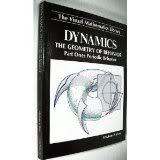I decided I needed to back-fill on the concept of state space
and the related concept of a phase plot.

So I read from Ralph Abraham and C. D. Shaw's so-called "chaos comix," Dynamics,
the Geometry of Behavior (1992), from book 1, pp.  1.1 - 2.4.7, which begins:

1.1 STATE SPACE

I wish I could lay my hands on one of the two four-volume sets that I own,
but they're in storage.  I suppose I'll quote it some other time.  Meanwhile,
to keep up with Abraham, check his web site at the Visual Math Institute.

( www.vismath.org

I went on to explain how Ordinary Differential Equations (ODEs) are
sorted out.  A single equation with one "variable" (actually a function) x(t)
will define the derivative of x(t) in terms of some function f of x.  If f
is linear then the equation is in principle solvable, and the
solution will be another function s(t).

x' = f(x)

A pair of ODEs can have two "variables," x(t) and y(t), and two equations defining
the two derivatives in terms of two functions f and g of x and y.  Again, if f and
g are linear then the set of equations tends to be solvable.

x' = f(x, y)
y' = g(x, y)

You will sometimes see higher order derivatives, like the second derivative of
y set to x, while the first derivative of x is some function of x and y.

y'' = x
x'  = f(x, y)

Or you may even see polynomial-like equations in which derivatives of different
orders have constant factors (shown here in caps) which can be easily put into
matrix form, allowing all sorts of tricks.

A y''' + B y'' + C y + D = 0

As always, here in the 21sty century the main issue is getting the equations right,
and then letting the computers solve them if possible or simulate them if not.

I showed how to solve a simple physics problem with these tools: the linear
spring.  This is kind of like the bowling ball hanging from a slinkey (or what
ever it was) in the Periodic Motions film.  Imagine that a spring provides force
in the opposite direction of its displacement with spring constant k, and it is
attached to mass m.  We have the force on the spring as:

f = -kx

and Newton's law:

f = ma

By algebra:

ma  = -kx
a   = -kx/m
x'' = -kx/m

(Note that x'' is another name for a, acceleration.)

Setting k and m to 1 for simplicity, and plugging this result into Wolfram Alpha,

x'' = -x

( www.wolframalpha.com/input/?i=x%27%27+%3D+-x )

Note that we get an equation, a graph, and a phase plot back.
Lesson 11 — 17 August 2010
concepts: Markov machine

( en.wikipedia.org/wiki/GNFA )

Markov tree

( en.wikipedia.org/wiki/Markov_chain )

In advance of this final lesson I made some notes as to what I wanted to cover,
including some things I missed, what they call "pickups" in filmmaking.

First I extended the idea of a finite state machine to include probability,
giving us the Markov machine.  A Markov machine without inputs
just transitions from state to state like an automaton, based on pre-established
probabilities.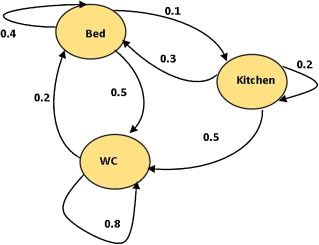Markov machine from "Computer
simulation of the activity of the elderly person
living independently in a Health Smart Home"
www.sciencedirect.com/science/article/pii/S0169260712001782

A Markov machine with inputs bases the probabilites on the state it is
in and the input it receives.

A technique developed by Dr. Markov is the Markov tree or chain.
You simply draw a tree of all possible outcomes after a certain number of steps,
and label each branch with the probablity of that transition.  To find the probablity
of a "leaf" on the tree, start at the root and multiply probabilities.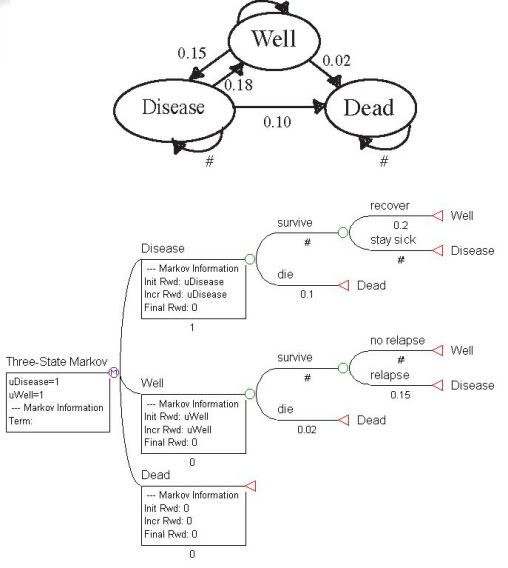Markov machine and tree tree from "Markov Models -
State Transition Models" by TreeAge Software
www.treeage.com/learnMore/MarkovModels.html

Next I trotted out the old "Monty Hall Problem" which causes so much confusion
and arguing.  The Wikipedia article explains it with a Markov tree.

( en.wikipedia.org/wiki/Monty_Hall_problem )

then from my blog on Eigenvectors (op. cit.), abd pages 2-3 of Garfinkel  (op. cit.).

I also wanted to show daughter some more detail of the interactive visualization
of the Lorenz Attractor and other state spaces.  I'd use the AVS5 software from
AVS Inc., and written a "plug-in" I called simply "system" in the C language.
I showed how I could make a change to the 3 ODEs in the code, defining variables
"x_dot," "y_dot" and "z_dot" in terms of x, y, z, and some constants on knobs.
Then I re-ran the visualization showing the change.

Winding up, I reviewed our list of goals from the first lesson and declared victory.
I closed with a look at the table from Bertalanffy's "General Systems Theory" (1968)
on the difficulty of solving equations.

( www.well.com/user/abs/equations.html )

This is the "dirty little secret" of Newton's scientific revolution: in most cases
we can write the equations but not solve them!  For four hundred years there was
practically a cover-up, until cheap computers and simulation came along to save us.

PostscriptJust got The Manga Guide to Linear Algebra (2012) today.

( www.amazon.com/exec/obidos/ASIN/1593274130/hip-20 )

I can hardly wait to read it.  I'm pretty sure I would've used it for the calculus
class if it had been available then...

Note Re:"If It's Just a Virtual Actor, Then Why
Am I Feeling Real Emotions?"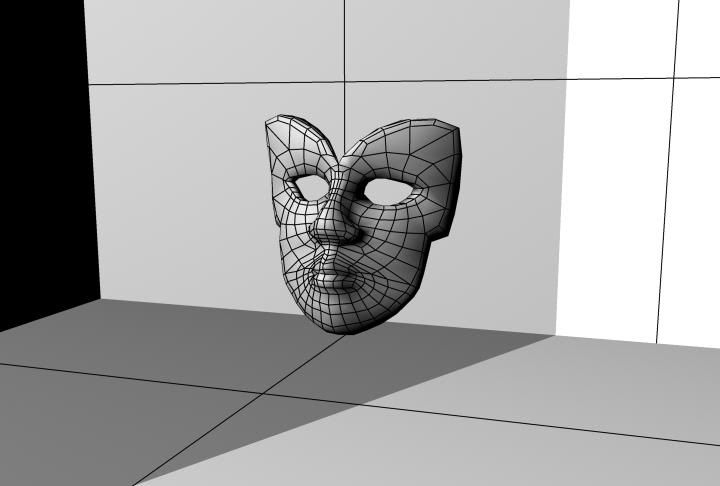Part five of this serialization will appear next time.

========================================================================
www.well.com/user/abs/Cyb/archive

========================================================================